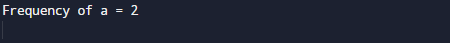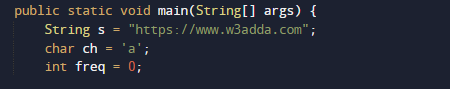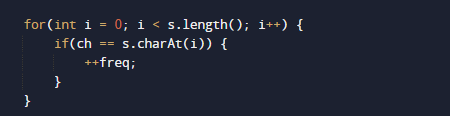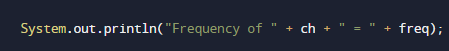# Java Program to Find the Frequency of Character in a String

In this tutorial you will learn about the Java Program to Find the Frequency of Character in a String and its application with practical example.

In this tutorial, we will learn to create a Program that will Find the Frequency of Character in a String using java programming.

## Prerequisites

Before starting with this program  we assume that you are best aware of the following Java programming topics:

• Java Operators.
• Basic input/output.
• Basic Java programming.
• Array and Multidimensional Array.
• Loops in python.
• String in python.
• Nested For loop.
• Class and object.

## What Is frequency of occurrence of a character?

Frequency means  to find and count  given  character present in the string. i.e Total number of occurrence of a particular character in a given string.

## Java Program to Find the Frequency of Character in a String

In this program we will find frequency of a given character will be calculated.First of all a string and a character to find the frequency of given character is to set.later we will find frequency of a given character in a string.

## OutputIn the above program, we have first declared and initialized a set variables required in the program.

• s = it will hold given string.
• ch = it will hold a finding character.
• i=  for iteration.
• freq= it will count total number of occurrence of a character.Values of string and a character will be assigned to variable ‘s’ and in ‘ch’ respectively. Now we will loop through the size of  a string using length() function . In each iteration we will compare string to given character to find the occurrence of a character and each matching condition we increase the counter value by  one as shown in below image.This process  will continue until loop ends , and at last we will print the total number occurrence of a given character.This is the process  of finding  the frequency of occurrence of a character in a String.

In this tutorial we have learn about the Java Program to Find the Frequency of Character in a String and its application with practical example. I hope you will like this tutorial.